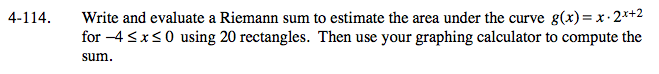### Home > CALC > Chapter Ch4 > Lesson 4.3.2 > Problem4-114

4-114.

Write and evaluate a Riemann sum to estimate the area under the curve g(x) = x · 2x + 2 for −4 ≤ x ≤ 0 using 20 rectangles. Then use your graphing calculator to compute the sum. Homework Help ✎Notice that the area will be negative.

Area ≈ -6.448
But you will need to show the setup and steps.

Use the eTool below to view the graph.
Click the link at right for the full version of the eTool: Calc 4-114 HW eTool